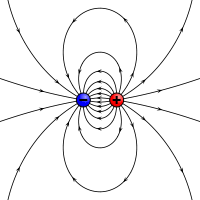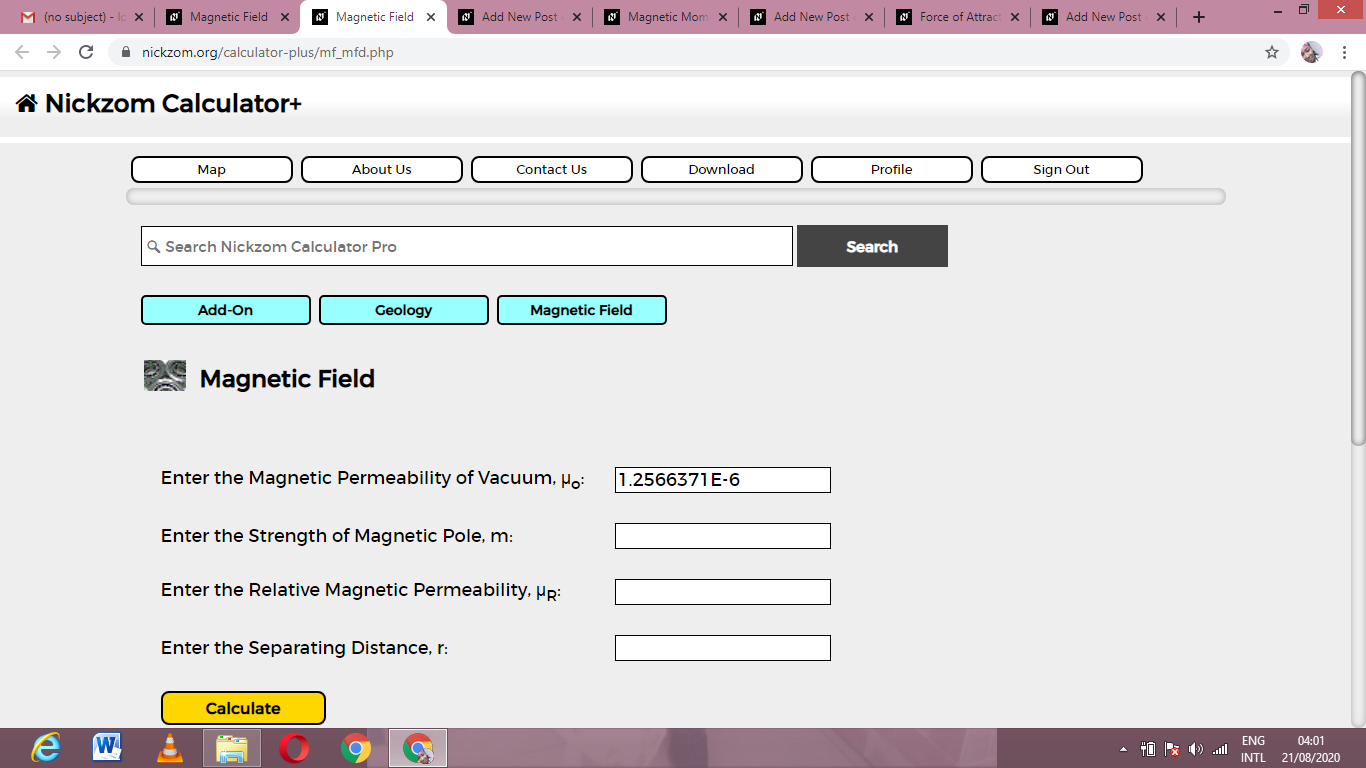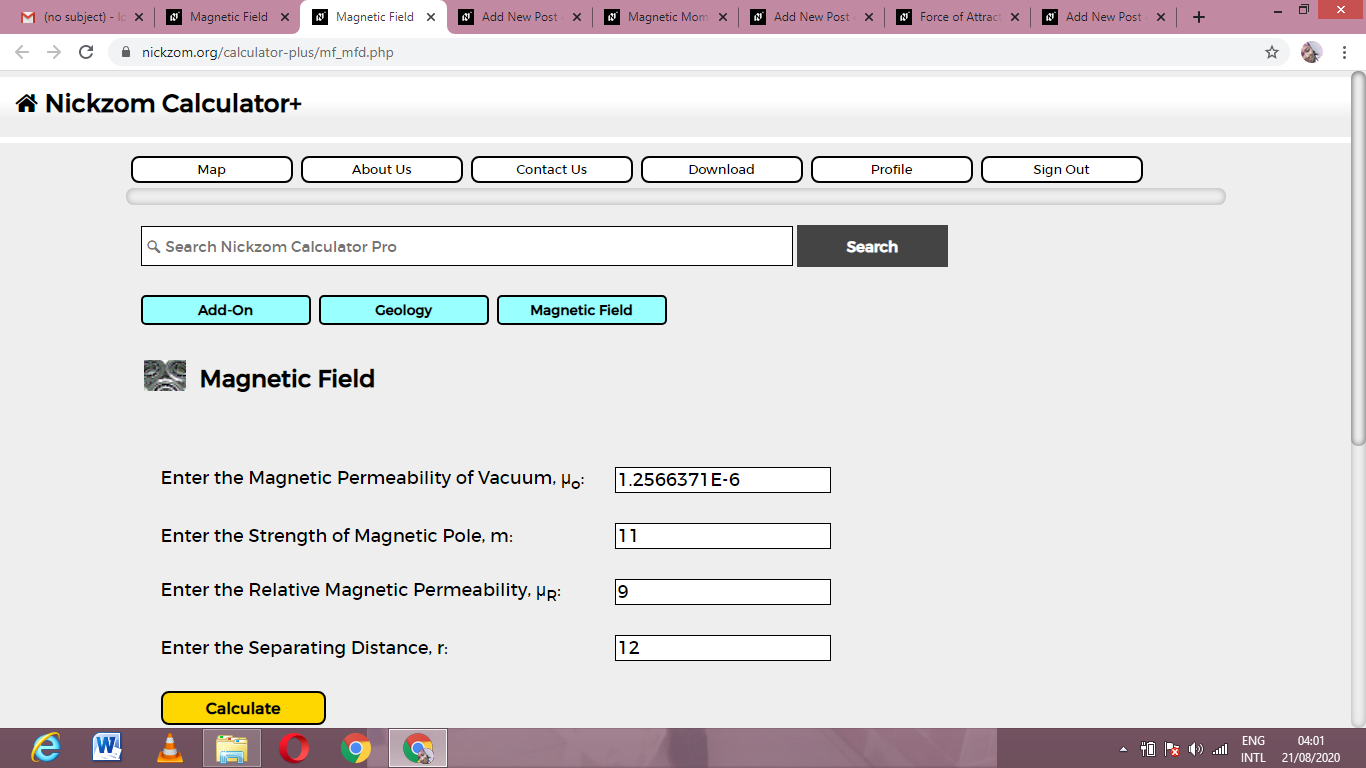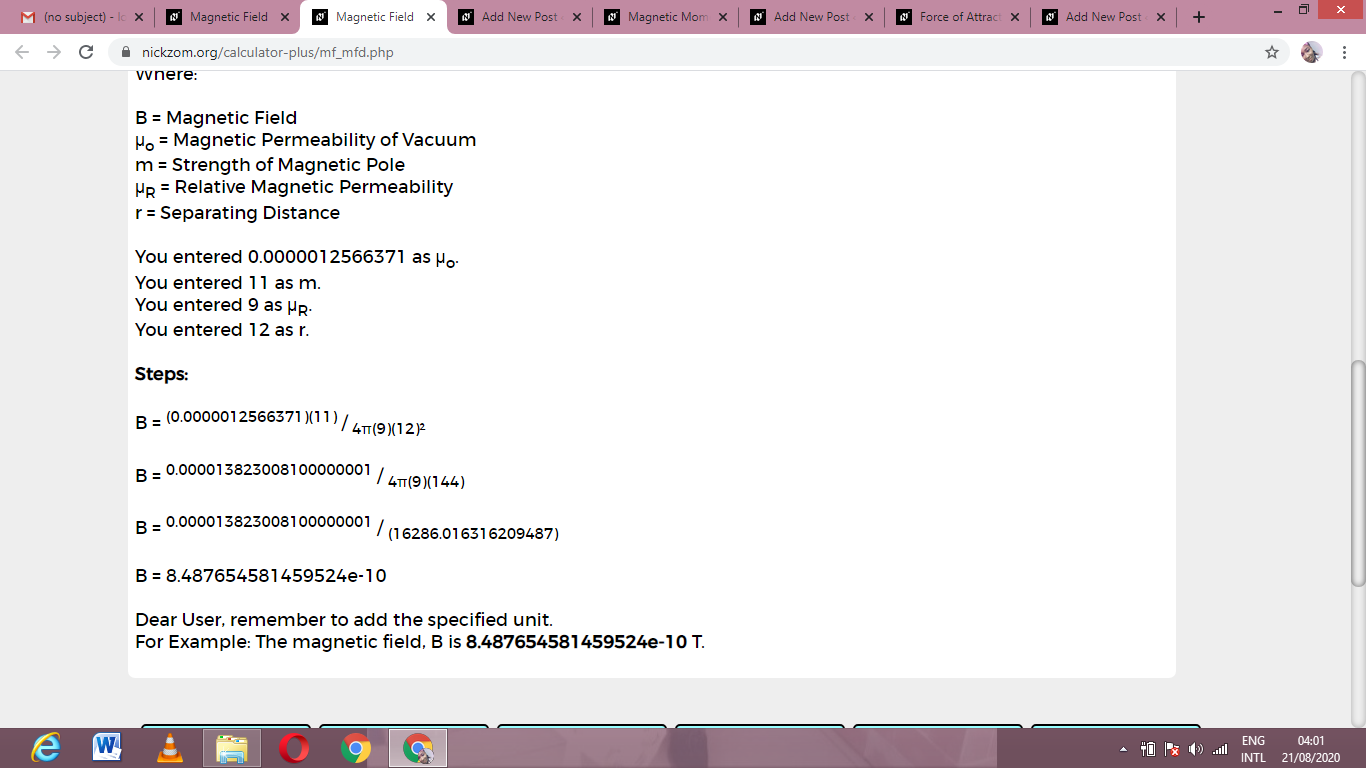# How to Calculate and Solve for Magnetic Field | Magnetic FieldThe image above represents magnetic field.

To compute for magnetic field, four essential parameters are needed and these parameters are Magnetic Permeability of Vacuum (μo), Strength of Magnetic Pole (m), Relative Magnetic Permeability (μRand Seperating Distance (r).

The formula for calculating magentic field:

B = μom / 4πμRr²

Where:

B = Magnetic Field
μo = Magnetic Permeability of Vacuum
m = Strength of Magnetic Pole
μR = Relative Magnetic Permeability
r = Separating Distance

Let’s solve an example;
Find the magnetic field when the magnetic permeability of vaccum is 1.2566371E-6, strength of magnetic pole is 11, relative magnetic permeability is 9 and the seperating distance is 12.

This implies that;

μo = Magnetic Permeability of Vacuum = 1.2566371E-6
m = Strength of Magnetic Pole = 11
μR = Relative Magnetic Permeability = 9
r = Separating Distance = 12

B = μom / 4πμRr²
B = (0.0000012566371)(11) / 4π(9)(12)²
B = 0.00001382 / 4π(9)(144)
B = 0.00001382 / (16286.0163)
B = 8.487e-10

Therefore, the magnetic field is 8.487e-10.

Nickzom Calculator – The Calculator Encyclopedia is capable of calculating the magnetic field.

To get the answer and workings of the magnetic field using the Nickzom Calculator – The Calculator Encyclopedia. First, you need to obtain the app.

You can get this app via any of these means:

To get access to the professional version via web, you need to register and subscribe for NGN 2,000 per annum to have utter access to all functionalities.
You can also try the demo version via https://www.nickzom.org/calculator

Apple (Paid) – https://itunes.apple.com/us/app/nickzom-calculator/id1331162702?mt=8
Once, you have obtained the calculator encyclopedia app, proceed to the Calculator Map, then click on Geology under Add-on.Now, Click on Magnetic Field under GeologyNow, Click on Magnetic Field under Magnetic FieldThe screenshot below displays the page or activity to enter your values, to get the answer for the magnetic field according to the respective parameters which are the Magnetic Permeability of Vacuum (μo), Strength of Magnetic Pole (m), Relative Magnetic Permeability (μRand Seperating Distance (r).Now, enter the values appropriately and accordingly for the parameters as required by the Magnetic Permeability of Vacuum (μo) is 1.2566371E-6, Strength of Magnetic Pole (m) is 11, Relative Magnetic Permeability (μRis 9 and Seperating Distance (r) is 12.Finally, Click on CalculateAs you can see from the screenshot above, Nickzom Calculator– The Calculator Encyclopedia solves for the magnetic field and presents the formula, workings and steps too.